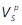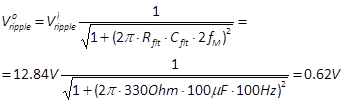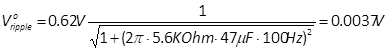The schema of the power supply unit for the stages of the amplifier is given in Figure 53. As discussed in Section 5.1, it is composed of a power supply transformer, a rectifier with its reservoir capacitor, and two sequences of RC smoothing filters, one per each channel of the stereo amplifier.

We start computing the voltage delivered by the rectifier via the reservoir capacitor, and its ripple voltage. We estimate the rating of the power transformer. Then, we compute the resistance of the smoothing filters. Finally, we compute the capacitances of the smoothing filters.

### 6.4.1    Voltage delivered by the rectifier and ripple voltage

The voltage produced by the rectifier via the reservoir capacitor, as discussed in Sections 5.1.5, depends on

• the type of rectifier,
• the mains frequency,
• the output voltage of the power transformer,
• the output impedance of the power transformer,
• the load seen by the reservoir capacitor,
• the capacitance of the reservoir capacitor

We will use a full wave rectifier and consider a mains frequency fM=50Hz. A 500V centre tapped power transformer has a peak voltage=250V∙1.414=353.5V. Suppose the output impedance of the power transformer is around Rs=27 Ohm, which is a realistic value.

The load seen by the reservoir capacitor is the impedance of the power supply filter plus the impedance of the entire amplifier. The impedance of the amplifier can be estimated by using the Ohm law, by dividing the voltage given to the first powered stage, which is the power stage, by the current absorbed by all stages. The quiescent current absorbed by the concertina and by the input stage was already discussed before and is respectively 0.98mA and 0.8mA. The quiescent current of the power stage is 28mA in each tube, which makes 56mA. When working in class A, the current absorbed by the power stage is always 56mA, since when one tubes conducts less the other conducts more. When delivering maximum output power, working in Class AB, the maximum absorbed current arrives up to 115mA. However, we will use Class A conditions to compute the expected DC voltage produced by the reservoir capacitor. So we use 56mA as a reference current for the power stage. This implies that when operating at maximum output power, the voltage delivered by the reservoir capacitor will be slightly lower. The total current absorbed by the amplifier is therefore 56mA+0.8mA+0.98mA=57.78mA. We have two channels so the total current should be doubled, obtaining IL=115.56mA. The voltage needed at the first powered stage, which is the power stage, is 300V. Therefore, the impedance of the amplifier is RL=300V/115,56mA=2.6k Ohm. We said that the total load seen by the reservoir capacitor is the impedance of the amplifier plus the impedance of the smoothing filter of the power stage. We will see that, given that the voltage delivered by the reservoir capacitor is close to what needed by the power stage, the impedance of the power stage smoothing filter is much smaller than the impedance of the amplifier, so we can ignore it and use only the impedance of the amplifier.

We now have all ingredients to estimate the voltage delivered by the reservoir capacitor. Suppose we use reservoir capacitor with a CR=22μF. The ratio RS/Ris 1.01% and 2πfMCRRL=18.4. According to the graph in Figure 39 we have a percentage Vdc/around 91%. The estimated DC output voltage at the reservoir capacitor is Vdc=352.5V∙91%≈321V.

Similarly, using the graph in Figure 40 we can determine the expected ripple voltage, ad discussed in Section 5.1.6. The green line, corresponding to RS/RL=1%, in correspondence of 2πfMCRRL=18.4, gives a ratio Vripple/Vdc around 4%. Therefore, the expected voltage ripple is Vripple=321∙4%=12.84V.

### 6.4.2    Rating of the power transformer

Using the parameters computed in previous section we can also compute the RMS current of the power transformer secondary, with the help of Figure 41. The red plot corresponding to RS/RL=1%, in correspondence of 2πfMCRRL=18.4, gives a ratio IS/IL around 1.6. Therefore the expected RMS current of the power transformer secondary is IS=115.56mA∙1.6=184.8mA. To guarantee safe operation we can chose a centre tapped 500V power transformer rated for a 350mA RMS current, or simply 500V C.T. @350mA.

### 6.4.3    Resistors of the smoothing filters

The two chain of filters, for the left and right channels of the amplifier, are identical, so we just need to compute values for one chain. Computation of the components of a smoothing filter was discussed in Section 5.1.8. We first compute the value of the resistors of the smoothing filters, going backward, from the last filter of the chain, which is the filter for the input stage.

In Section 6.2.2, we determined that the anode voltage of the 12AX7 vacuum tube of the input stage is 280V. Its quiescent current is 0.8mA. When delivering maximum power, the current varies +/- 0.05mA from the quiescent current and has little impact, so we can safely use the quiescent current in our computation. We also determined that the voltage required by the anode of the 12AX7 vacuum tube of the next stage, the concertina phase splitter, is 290V. As explained in Example 21, the resistor of the smoothing filter between the concertina and the input stage has to produce a voltage drop of 10V. Given that the current traversing the resistor is 0.8mA, we have that its resistance must be 10V/0.8mA=12.5K Ohm. The closest standard is 12K Ohm, which is close to the needed value. The power dissipated by the resistor is 10V∙0.8mA=0.008W. Therefore, we can use a resistor rated for 0.5W.

The voltage of the anodes of the EL84 vacuum tubes is 300V. The current absorbed by the concertina at its quiescent state is 0.98mA. At maximum power, the current varies +/- 0.18mA, with respect to the quiescent state. Also in this case, the variation does not affect significantly our computation, and we use the quiescent current as reference. To obtain the needed 290V at the concertina, the resistor of the smoothing filter between the power stage and the concertina has to produce a voltage drop of 10V. The current traversing this resistor is the current absorbed by the input stage and that absorbed by the concertina, that is 0.8mA+0.98mA=1.78mA. The value of the resistor is 10V/1.78mA=5.6K. The power dissipated by the resistor is 0.018W. Also in this case we case we can use one rated for 0.5W.

Finally, we compute the resistor of the smoothing filter of the power stage. It should drop 21V, from the 321V delivered by the reservoir capacitor, to obtain the wanted 300V. The current traversing the resistor is the sum of the current absorbed by the power stage, the concertina, and the input stage, that is 56mA+0.8mA+0.98mA=58mA.  The value of the needed resistance is 21V/58mA=362 Ohm. The closeststandard is 330 Ohm. The power dissipated by the resistor is 1.34W, so it is convenient to choose one rated for 5W. The computed resistance is much smaller than the impedance of the amplifier. Therefore, as we said before, adding it, to exactly compute the total load seen by the reservoir capacitor, does not affect significantly our estimation.

### 6.4.4    Capacitors of the smoothing filters

We can now chose the values of the capacitors of the smoothing filters. In section 6.4.1 we computed that the ripple voltage after the reservoir capacitor is 12.84V. The DC voltage of the power stage is 300V, so this ripple voltage is around 4% of DC voltage. Recommended ripple voltage in a push-pull stage is around 0.5% to 2% (see Section 5.1.8)  so we need to significantly attenuate it.  If the capacitor of the smoothing filter of the power stage is 100μF, according to Section 5.1.8, we have that the voltage ripple is.

This corresponds to 0.2% of the power stage DC voltage, so a very good value.

Following the same procedure, we chose the value of the capacitor of the smoothing filter of the phase splitter stage. With a capacitor of 47μF, we have thatThis corresponds to 0.001% of the phase splitter DC voltage, which is 290V, and lower than acceptable values, which are around 0.01% to 0.05%.

Finally, we set the value of the capacitor of the smoothing filter of the input stage. With a capacitor of 4.7μF, we have thatThe DC voltage of the input stage is 280V. The ripple voltage corresponds to 0.00003% of the DC voltage. Ripple voltage percentage is, also in this case, smaller than safe values, which are around 0.001% to 0.002%.